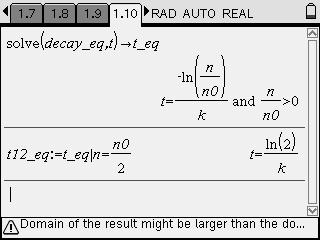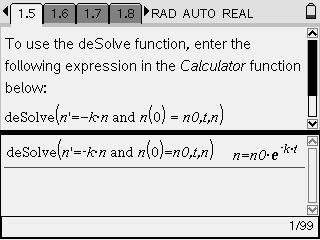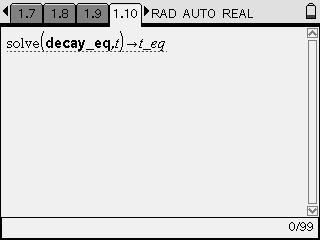# Activities

••• ##### Subject Area

• Science: Physics: Atomic Physics

• ##### Author9-12

15 Minutes

• ##### Device
• TI-Nspire™ CAS
• ##### Software

TI-Nspire™ CAS

1.7

• ##### Other Materials
• pen or pencil
• blank sheet of paper
• ##### Report an Issue

Bell Ringer: The Radioactive Decay Equations#### Activity Overview

Students derive the equation for the number of parent atoms remaining as a function of time. They rearrange the equation to find the equation for half-life.

#### Key Steps

•Students derive the equations describing radioactive decay.

They begin by solving the differential equation that relates time (t), the number of parent isotopes at that time (n), the decay constant (k), and the initial number of parent isotopes (n0).

•They rearrange the equation to solve for t.

•They solve this equation to determine the equation for the half-life of the isotope.

They then use the half-life equation to solve several problems.